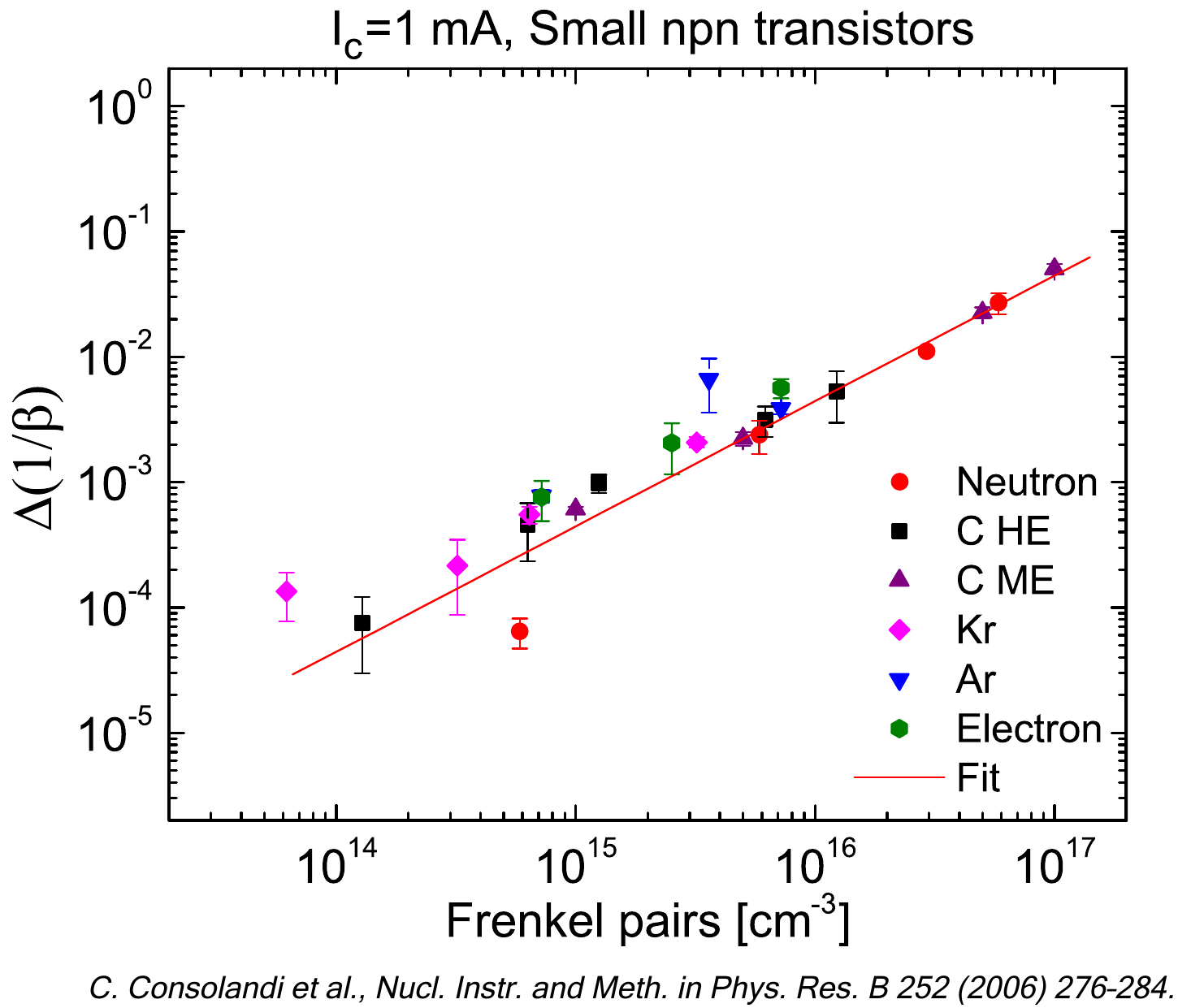SR-NIEL – 7 Screened Relativistic (SR) Treatment for NIEL Dose Nuclear and Electronic Stopping Power Calculator (version 10.11)This is the SR-NIEL Code Web application. Registered users can acess NIEL calculators with a) Robinson and b) Akkerman partition functions.

- Electrons NIEL Calculator (registered)

- Protons & Ions NIEL Calculator (registered)

### How to use this NIEL Calculator

This tool calculates the Screened Relativistic (SR) Non-Ionizing Energy Loss (NIEL) curve for a particle incident on a material. Information about the computation method is available at look at the NIEL and Displacement Stopping Power page.

The input parameters and options for the tool are described below. When the input form has been completed, pressing the "CALCULATE" button will start the calculation and open the "Results" page (allow for pop-up in your browser settings). The result page will be also linked at the bottom of the calculator page.

### Input Parameters:

- Incident particle (for Protons&Ions Calculator)
- Proton and ion model (for Protons&Ions Calculator)
- Electron form (for Electrons Calculator)
- Partition Function
- Target material
- Calculator energy limits
- Particle Fluence for Dose calculation.

### Incident Particle

In the Protons&Ions Calculator, using the pull down menu, the user can select the species of the incident particle, either a proton or one of the elemental ions.

### Electron Form Factor

In the Electron Calculator, the user can select the nuclear form factor (Exponential, Gaussian or Uniform ) model to be used. The form factor accounts for the spatial distribution of charge density probed in the electron-nucleus scattering.

### Proton and Ion Model

When a proton or ion is selected as incident particle, it is possible to specify the different contribution to the total non-ionizing energy loss that is returned: the Coulomb scattering contribution, the contribution of the Hadronic interaction or the Total contribution (Hadronic + Coulomb).

In the present NIEL calculators, for protons up to 1 GeV and alpha-particles up to 1 GeV/nucleon impinging on elements with atomic number from Z=4 up to Z=51, as already mentioned in NIEL and Displacement Stopping Power page, the hadronic contributions were interpolated or extrapolated from those obtained from [Jun et al. (20032004),Jun (2017)] regarding 10 absobers, i.e., C, Al, Si, P, Ga, Ge, As, In, Cu, Se with corresponding displacement thresold energy of 35, 20, 21, 9, 10, 20, 10, 7, 25, 20 eV, respectively .For silicon absorbers, in addition, the hadronic contribution for energies from 1 up to 24 GeV was obtatined from [Huhtinen (2002)].
As default option (no scaling), no Ed dependence is included for the hadronic contribution to NIEL. It has to be remarked that such a beviour is consistent with the results obtained for neutron damage functions with MeV neutrons (up to 20 MeV) by means of NJOY-2012 code (e.g. see NJOY based neutron NIEL calculator). As alternative option (linearly scaled), the hadronic contribution are linearly varied with respect to the calculated by the same amount found for Coulomb contributions.

### Partition Function

The selection allows one to specify the analitycal approximation of the partition function used in the NIEL calculation. Registered users can select Robinson or Akkermann approximation. More information is available in the Energy Partition Function page.

### Target Material

In this section it is possible to specify the composition of the target material selecting the number of elements in the compound. The required parameters for each element are:

- Atomic number (Z)/Chemical symbol
- Stoichiometric index or element fraction
- The displacement threshold energy [eV]

Experimentally determined Ed values:

• Ga: 21.5 eV, As: 21.5 eV as reported in [Campesato et al. (2019)] from proton, electron and neutron irradiations of solar cells. In Triple Junction solor cells, in which the dominat damage mechanism is that one due to the GaAs middle junction, a commonly effective value of Ed was 24 eV [Campesato et al. (2018)], [Campesato et al. (2019)].
• Ge: 40.5 eV as reported in [Campesato et al. (2019)].
• In: 43 eV, Ga: 21 eV, P: 21 eV as reported in [Campesato et al. (2018)] for In0.49Ga0.51P single junction solar cells irradiated with electrons and protons. In [Campesato et al. (2019)] with electron, proton and neutron irradiation, it was shown that a commonly effective value of Ed=38 eV can be employed in an equivalent way.

### Energy Limits

This section define the energy limits of the calculation, and the following parameters will be defined:
- Minimum Energy of the incident particle
- Maximum Energy of the incident particle

The output table will contain 20 fixed values for each decade within the above selected range.

Moreover a text box is provided for entering additional energies. The input format is one energy per line; it is also possible to copy and paste energy values.

if Hadronic calculation is included (Hadron only and Coulomb+Hadron cases) the Maximum energy is limited to 1 GeV/nucleon (due to hadronic interaction data availability); for proton in silicon absorbers the Maximum energy is limited at 24 GeV.

### Particle Fluence for Displacement Damage Dose Calculation

Here is defined the particle fluence that is used to obtain the deposited NIEL Dose (i.e., the displacement damage dose).

### Result

The result page contains the input parameters, the NIEL curve and the result table, which includes the NIEL, and the NIEL Dose (in [MeV g-1] and [Gy])